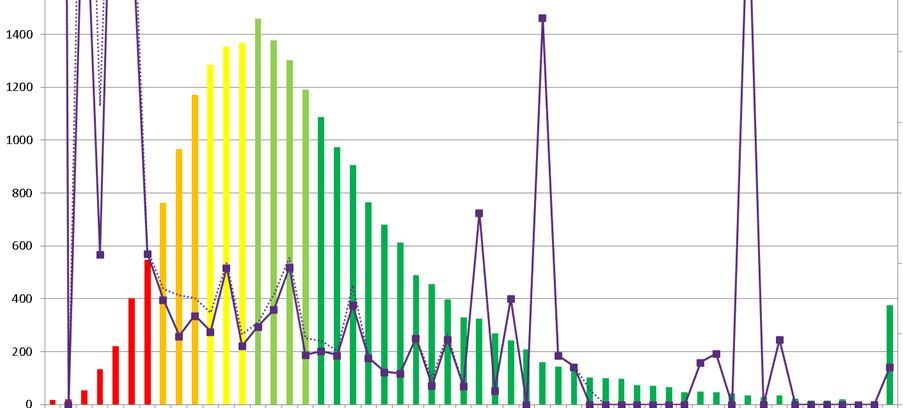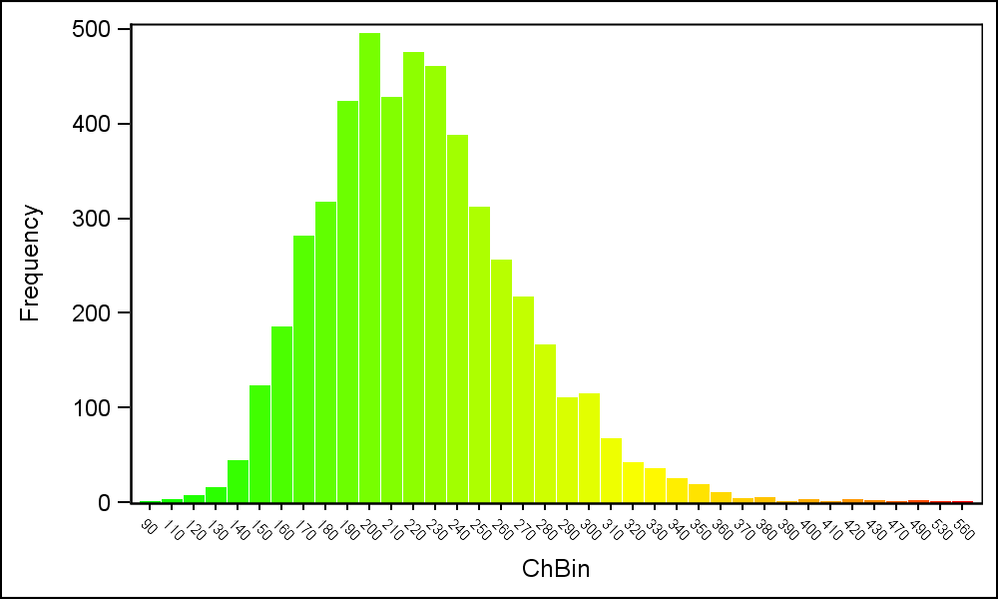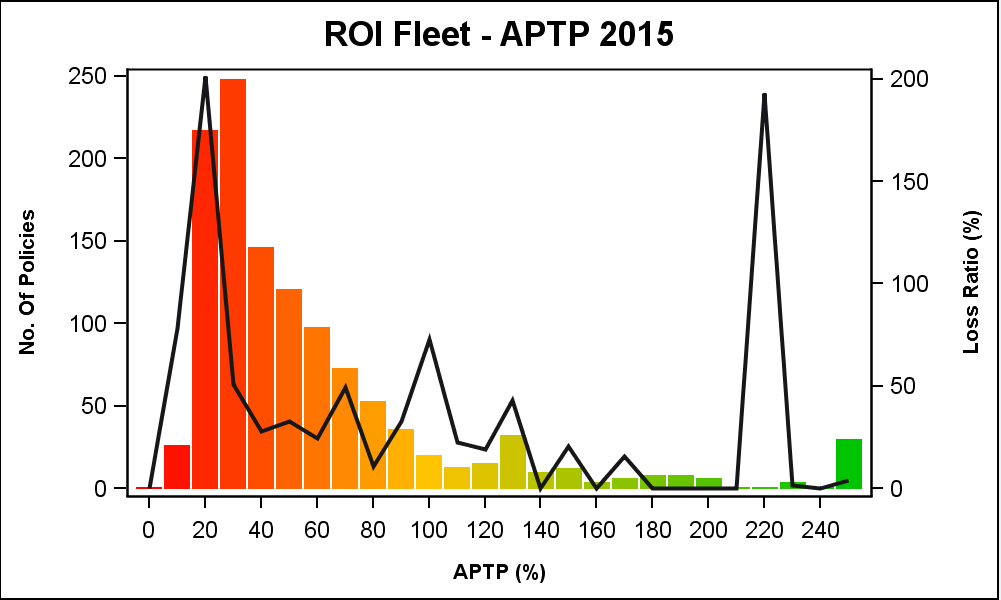## Varying colours on histogram

Hi

Is it possible to have a different colours accross a single histogram? Ideally I would like the bars on the left to be green and for the colours to gradually move from green to red. I have attached an example of what I would like (produced in excel). I have SAS/Graph 9.3. Here is my code below.

proc sgplot data=test ;
title bold height = 1.5 "APTP";
vbar level / response=polexp fillattrs=(color = deppk ) legendlabel =  "exposure years";
yaxis label = "No. Of Policies" labelattrs=(weight=bold size = 10);
xaxis display = (nolabel)  ;
run;1 ACCEPTED SOLUTION

Accepted SolutionsJay54
Meteorite | Level 14

## Re: Varying colours on histogram

Your data has the % sign in it.  I stripped it out and used part of your data.  I made a small change to colors to get a more pleasing results.

%let gpath='.';
%let dpi=200;
ods html close;
ods listing gpath=&gpath image_dpi=&dpi;

data APTP;
input aptp @@;
datalines;
146.9 162.6 131.3 106.6 111.6 115.6 267.3 104.6 181.8 134.7
58.23 77.17 181.5 121.9 144.0 63.95 89.91 160.4 102.4 134.3
21.35 27.43 17.63 16.47 28.37 25.72 19.63 40.04 41.38 24.32
21.68 19.24 19.35 39.85 19.47 35.39 18.56 35.69 31.12 24.04
30.93 29.48 29.77 59.55 22.29 27.22 42.56 26.85 13.35 24.43
31.49 21.18 29.99 8.67 15.16 24.71 23.93 20.14 23.56 35.93
29.96 29.11 23.78 61.96 35.04 19.63 23.22 21.45 21.45 23.07
15.94 56.22 31.74 33.38 21.45 66.18 34.03 23.22 23.51 21.55
21.34 24.22 22.28 36.76 20.57 50.15 35.09 45.70 22.27 22.85
41.69 22.95 26.14 29.48 36.37 25.44 28.40 11.71 23.45 54.05
41.19 20.83 22.36 17.32 24.94 24.72 30.65 45.35 29.96 22.75
36.14 38.45 36.08 22.55 26.93 58.45 21.87 39.16 22.72 45.48
26.82 26.70 23.61 23.77 48.90 24.83 29.12 23.07 29.46 22.63
246.0 44.67 20.73 23.08 27.95 24.94 52.23 23.61 26.75 34.98
21.90 24.99 24.58 25.28 45.86 27.84 31.23 22.85 63.76 97.93
22.54 22.32 29.01 39.96 62.94 21.55 17.16 31.01 31.99 26.82
39.59 25.49 33.89 27.50 50.05 45.05 71.57 75.29 40.84 78.90
36.86 69.20 62.79 41.39 47.68 101.4 37.80 43.31 31.75 55.30
47.87 39.63 36.83 38.74 34.37 40.86 47.75 39.54 43.79 81.32
35.92 46.10 26.07 28.78 41.59 43.69 45.33 51.00 40.94 43.83
35.51 37.11 34.54 37.31 40.44 35.56 71.05 22.52 46.78 48.84
;
run;

/*proc sgplot data=aptp;*/
/*histogram aptp;*/
/*run;*/

data aptp2;
retain BinInt 5 maxbin 0 minbin 1e6;
set aptp end=last;
label bin='APTP';

if aptp ne . then bin=BinInt*floor(aptp/BinInt);
minbin=min(minbin, bin);
maxbin=max(maxbin, bin);
if last then do;
call symput("MinBin", minbin);
call symput("MaxBin", maxbin);
call symput("BinInt", BinInt);
end;
run;

%put "Min Bin = &MinBin";
%put "Max Bin = &MaxBin";
%put "Bin Int = &BinInt";

/*--Define attributes map data set--*/
data AttrMap;
length FillColor \$8 LineColor \$8;
id='Hist';
ghigh=196; /*--High value for Green--*/
rhigh=255; /*--High value for Red--*/

mid=(&minbin + &maxbin) / 2;
LineColor='CX000000';

do val=&minbin to &maxbin by &BinInt;
value=put(val, 5.0);
if val < mid then do;
g=ghigh; b=0; r=rhigh*(val-&minbin)/ (mid-&minbin);
end;
else do;
r=rhigh; b=0; g=ghigh*(1-((val-&minbin) - (mid-&minbin))/ (mid-&minbin));
end;
fillcolor='CX' || put(r, hex2.) || put(g, hex2.) || put(b, hex2.);
output;
end;
run;

proc print;run;

/*--Graph--*/
ods graphics / reset width=5in height=3in imagename='Color_Resp_Histogram_APTP';
proc sgplot data=aptp2 dattrmap=AttrMap noautolegend;
vbar bin / barwidth=0.9 group=bin attrid=Hist nooutline;
xaxis valueattrs=(size=6) fitpolicy=thin;
run;11 REPLIES 11

## Re: Varying colours on histogram

I dont think so , use Bar Plot there you can change it ..

## Re: Varying colours on histogram

Could you advise how this would be done. If I had 50 levels would I need to code in the colour of each level (bar) indiviudally?

Thank youDanH_sas
SAS Super FREQ

## Re: Varying colours on histogram

I can't see your data, and I'm not sure about your SAS version, but try adding COLORRESPONSE=level on your VBAR statement and see if that gives you what you want.Jay54
Meteorite | Level 14

## Re: Varying colours on histogram

COLORRESPONSE is available with SAS 9.40M3  For reference, see:  http://blogs.sas.com/content/graphicallyspeaking/2015/09/21/response-colors-and-thickness/

With SAS 9.3, one way this could be done is by using a Discrete Attributes map.  You would have to set group values, say 1-50 for the 50 bars.  Then, define a DATTRMAP data set that will assign the required shade of the color to each group value.  Then, you can use this DATTRMAP data set with the ATTRID to color each bar.  Note:  Since the DATTRMAP is set to a data set, the large number of entries with color shade can be created programmatically.

Here is an article on using the Discrete Attributes Map.  While the example uses a Series plot, the same will apply to a bar chart.

http://blogs.sas.com/content/graphicallyspeaking/2013/04/02/attribute-maps-1/

## Re: Varying colours on histogram

Hi Sanjay, thanks for this - I will be using the same graph each month so this approach would be fine. I'm not sure how it would work though if I have 54 levels (called "1", "2","3", etc.) how do I define a colour for each?

I have attached my dataset.Jay54
Meteorite | Level 14

## Re: Varying colours on histogram

Here is a sample program.

%let gpath='.';
%let dpi=200;
ods html close;
ods listing gpath=&gpath image_dpi=&dpi;

data heart;
retain BinInt 10 maxbin 0 minbin 1e6;
set sashelp.heart(keep=Cholesterol Systolic) end=last;

if cholesterol ne . then ChBin=BinInt*floor(cholesterol/BinInt);
minbin=min(minbin, chbin);
maxbin=max(maxbin, chbin);
if last then do;
call symput("MinBin", minbin);
call symput("MaxBin", maxbin);
call symput("BinInt", BinInt);
end;
run;

/*--Define attributes map data set--*/
data AttrMap;
length fillcolor \$8;
id='Hist';

mid=(&minbin + &maxbin) / 2;

do val=&minbin to &maxbin by &BinInt;
value=put(val, 5.0);
if val < mid then do;
g=255; b=0; r=255*(val-&minbin)/ (mid-&minbin);
end;
else do;
r=255; b=0; g=255*(1-((val-&minbin) - (mid-&minbin))/ (mid-&minbin));
end;
fillcolor='CX' || put(r, hex2.) || put(g, hex2.) || put(b, hex2.);
output;
end;
run;

/*--Graph--*/
ods graphics / reset width=5in height=3in imagename='Color_Resp_Histogram';
proc sgplot data=heart dattrmap=AttrMap noautolegend;
vbar chbin / barwidth=0.9 group=chbin attrid=Hist nooutline;
xaxis valueattrs=(size=6);
run;## Re: Varying colours on histogram

Hi Sanjay

Thank you for your very helpful reply. Unfortunately I've been working on adjusting your code for the past 2 hours! to my own dataset (attached - just one column of data) but I just can't get it to work. I'm quite new to SAS/Graph so apologies for this.

I have attached my dataset. I want it to look the same as your historgram (green to red). The difference is that my dataset contains percentages and the intervals should be 5% or 0.05. My code is below:

I'm losing it here!!

data aptp;
retain BinInt 0.05 maxbin 0 minbin 1e6;
set aptp.Tariffprem_pol6(keep=APTP) end=last;

if APTP ne . then ChBin=min(BinInt*floor(APTP/BinInt),3);
minbin=min(minbin, chbin);
maxbin=max(maxbin, chbin);
if last then do;
call symput("MinBin", minbin);
call symput("MaxBin", maxbin);
call symput("BinInt", BinInt);
end;
run;

data AttrMap;
length fillcolor \$8;
id='Hist';

mid=(&minbin + &maxbin) / 2;

do val=&minbin to &maxbin by &BinInt;
value=put(val, 5.0);
if val < mid then do;
g=255; b=0; r=255*(val-&minbin)/ (mid-&minbin);
end;
else do;
r=255; b=0; g=255*(1-((val-&minbin) - (mid-&minbin))/ (mid-&minbin));
end;
fillcolor='CX' || put(r, hex2.) || put(g, hex2.) || put(b, hex2.);
output;
end;
run;

ods graphics / reset width=5in height=3in imagename='Color_Resp_Histogram';
proc sgplot data=aptp dattrmap=AttrMap noautolegend;
vbar chbin / barwidth=0.9 group=chbin attrid=Hist nooutline;
xaxis valueattrs=(size=6);
run;Jay54
Meteorite | Level 14

## Re: Varying colours on histogram

Your data has the % sign in it.  I stripped it out and used part of your data.  I made a small change to colors to get a more pleasing results.

%let gpath='.';
%let dpi=200;
ods html close;
ods listing gpath=&gpath image_dpi=&dpi;

data APTP;
input aptp @@;
datalines;
146.9 162.6 131.3 106.6 111.6 115.6 267.3 104.6 181.8 134.7
58.23 77.17 181.5 121.9 144.0 63.95 89.91 160.4 102.4 134.3
21.35 27.43 17.63 16.47 28.37 25.72 19.63 40.04 41.38 24.32
21.68 19.24 19.35 39.85 19.47 35.39 18.56 35.69 31.12 24.04
30.93 29.48 29.77 59.55 22.29 27.22 42.56 26.85 13.35 24.43
31.49 21.18 29.99 8.67 15.16 24.71 23.93 20.14 23.56 35.93
29.96 29.11 23.78 61.96 35.04 19.63 23.22 21.45 21.45 23.07
15.94 56.22 31.74 33.38 21.45 66.18 34.03 23.22 23.51 21.55
21.34 24.22 22.28 36.76 20.57 50.15 35.09 45.70 22.27 22.85
41.69 22.95 26.14 29.48 36.37 25.44 28.40 11.71 23.45 54.05
41.19 20.83 22.36 17.32 24.94 24.72 30.65 45.35 29.96 22.75
36.14 38.45 36.08 22.55 26.93 58.45 21.87 39.16 22.72 45.48
26.82 26.70 23.61 23.77 48.90 24.83 29.12 23.07 29.46 22.63
246.0 44.67 20.73 23.08 27.95 24.94 52.23 23.61 26.75 34.98
21.90 24.99 24.58 25.28 45.86 27.84 31.23 22.85 63.76 97.93
22.54 22.32 29.01 39.96 62.94 21.55 17.16 31.01 31.99 26.82
39.59 25.49 33.89 27.50 50.05 45.05 71.57 75.29 40.84 78.90
36.86 69.20 62.79 41.39 47.68 101.4 37.80 43.31 31.75 55.30
47.87 39.63 36.83 38.74 34.37 40.86 47.75 39.54 43.79 81.32
35.92 46.10 26.07 28.78 41.59 43.69 45.33 51.00 40.94 43.83
35.51 37.11 34.54 37.31 40.44 35.56 71.05 22.52 46.78 48.84
;
run;

/*proc sgplot data=aptp;*/
/*histogram aptp;*/
/*run;*/

data aptp2;
retain BinInt 5 maxbin 0 minbin 1e6;
set aptp end=last;
label bin='APTP';

if aptp ne . then bin=BinInt*floor(aptp/BinInt);
minbin=min(minbin, bin);
maxbin=max(maxbin, bin);
if last then do;
call symput("MinBin", minbin);
call symput("MaxBin", maxbin);
call symput("BinInt", BinInt);
end;
run;

%put "Min Bin = &MinBin";
%put "Max Bin = &MaxBin";
%put "Bin Int = &BinInt";

/*--Define attributes map data set--*/
data AttrMap;
length FillColor \$8 LineColor \$8;
id='Hist';
ghigh=196; /*--High value for Green--*/
rhigh=255; /*--High value for Red--*/

mid=(&minbin + &maxbin) / 2;
LineColor='CX000000';

do val=&minbin to &maxbin by &BinInt;
value=put(val, 5.0);
if val < mid then do;
g=ghigh; b=0; r=rhigh*(val-&minbin)/ (mid-&minbin);
end;
else do;
r=rhigh; b=0; g=ghigh*(1-((val-&minbin) - (mid-&minbin))/ (mid-&minbin));
end;
fillcolor='CX' || put(r, hex2.) || put(g, hex2.) || put(b, hex2.);
output;
end;
run;

proc print;run;

/*--Graph--*/
ods graphics / reset width=5in height=3in imagename='Color_Resp_Histogram_APTP';
proc sgplot data=aptp2 dattrmap=AttrMap noautolegend;
vbar bin / barwidth=0.9 group=bin attrid=Hist nooutline;
xaxis valueattrs=(size=6) fitpolicy=thin;
run;## Re: Varying colours on histogram

Many thanks Sanjay.that really helps

I've included a secondary axis which shows the the loss ratio. It took me a while (and the approach is probably far from optimal as i get an error when i run the code) but it does work (picture of graph attached).A challenge was that I wanted the loss ratio (claims (tot_inc) divided by premium (nep)) for each aptp band. So the only way I could get this to work was to calulcate a loss ratio each aptp group, then divide it by the number of policies in that aptp interval and use a stat=sum in the sgplot procedure. It works but I get this warning:

WARNING: Once a GROUP variable is used in a categorical chart, that

GROUP variable must be used in all overlaid charts. The

specified GROUP variable has been removed from the graph

display.

Anyway, (this isn't crucial) but I would like there to be a legend so that the user knows which graph relates to what axis. The vbar legend gives a description for every level (26 levels) which is not what I want - ideally i'd like one legend to explain the histogram i.e. a "Bars show exposure dist". Otherwise I could include a legend just for my vline graph (loss ratio) but not sure if this is possible.

Also, is it possible to reformat so the two y axis' give percentages.

Here's my full code now and I've attached the dataset i'm using.

%let gpath='.';

%let dpi=200;

ods html close;

ods listing gpath=&gpath image_dpi=&dpi;

data test(keep = aptp2 tot_inc nep);

set TariffPrem_pol5;

run;

data aptp2;

retain BinInt 10 maxbin 0 minbin 1e6;

set TariffPrem_pol5 end=last;

label bin='APTP';

if aptp2 ne . then bin=BinInt*floor(aptp2/BinInt);

minbin=min(minbin, bin);

maxbin=max(maxbin, bin);

if last then do;

call symput("MinBin", minbin);

call symput("MaxBin", maxbin);

call symput("BinInt", BinInt);

end;

run;

proc summary data = aptp2 nway missing;

class bin;

var tot_inc nep;

output out = lossratio(drop = _type_) sum=;

run;

data lossratio1 (keep = bin loss_ratio);

set lossratio;

format loss_ratio 8.2;

loss_ratio = (int((tot_inc/nep)*10000)/100)/_freq_;

run;

proc sort data = lossratio1; by bin; run;

proc sort data = aptp2; by bin; run;

data aptp2;

merge aptp2 lossratio1;

by bin;

run;

%put "Min Bin = &MinBin";

%put "Max Bin = &MaxBin";

%put "Bin Int = &BinInt";

/*--Define attributes map data set--*/

data AttrMap;

length FillColor \$8 LineColor \$8;

id='Hist';

ghigh=196; /*--High value for Green--*/

rhigh=255; /*--High value for Red--*/

/*mid=(&minbin + &maxbin) / 2;*/

mid = 100; /* set 100% APTP as neutral point */

LineColor='CX000000';

do val=&minbin to &maxbin by &BinInt;

value=put(val, 5.0);

if val < mid then do;

r=rhigh; b=0; g=ghigh*(val-&minbin)/ (mid-&minbin);

end;

else do;

g=ghigh; b=0; r=rhigh*(1-((val-&minbin) - (mid-&minbin))/ (&maxbin-mid));

end;

fillcolor='CX' || put(r, hex2.) || put(g, hex2.) || put(b, hex2.);

output;

end;

run;

/*--Graph--*/

ods graphics / reset width=5in height=3in imagename='Color_Resp_Histogram_APTP';

proc sgplot data=aptp2 dattrmap=AttrMap noautolegend;

title bold height = 1.5 "ROI Fleet - APTP 2015";

vbar bin / barwidth=0.9 group=bin attrid=Hist nooutline ;

vline bin / response = loss_ratio stat=sum y2axis lineattrs=(color=blb thickness = 2) legendlabel = "Loss Ratio" ;

yaxis label = "No. Of Policies" labelattrs=(weight=bold size = 8);

y2axis label = "Loss Ratio (%)" labelattrs=(weight=bold size = 8);

xaxis label = "APTP (%)" labelattrs=(weight=bold size = 8) fitpolicy=thin;

run;

## Re: Varying colours on histogram

Many thanks Sanjay.that really helps

"I've included a secondary axis which shows the the loss ratio. It took me a while (and the approach is probably far from optimal as i get an error when i run the code) but it does work (picture of graph attached).A challenge was that I wanted the loss ratio (claims (tot_inc) divided by premium (nep)) for each aptp band. So the only way I could get this to work was to calulcate a loss ratio each aptp group, then divide it by the number of policies in that aptp interval and use a stat=sum in the sgplot procedure. It works but I get this warning:

WARNING: Once a GROUP variable is used in a categorical chart, that

GROUP variable must be used in all overlaid charts. The

specified GROUP variable has been removed from the graph

display.

Anyway, (this isn't crucial) but I would like there to be a legend so that the user knows which graph relates to what axis. The vbar legend gives a description for every level (26 levels) which is not what I want - ideally i'd like one legend to explain the histogram i.e. a "Bars show exposure dist". Otherwise I could include a legend just for my vline graph (loss ratio) but not sure if this is possible.

Also, is it possible to reformat so the two y axis' give percentages.

Here's my full code now and I've attached the dataset i'm using.

%let gpath='.';

%let dpi=200;

ods html close;

ods listing gpath=&gpath image_dpi=&dpi;

data test(keep = aptp2 tot_inc nep);

set TariffPrem_pol5;

run;

data aptp2;

retain BinInt 10 maxbin 0 minbin 1e6;

set TariffPrem_pol5 end=last;

label bin='APTP';

if aptp2 ne . then bin=BinInt*floor(aptp2/BinInt);

minbin=min(minbin, bin);

maxbin=max(maxbin, bin);

if last then do;

call symput("MinBin", minbin);

call symput("MaxBin", maxbin);

call symput("BinInt", BinInt);

end;

run;

proc summary data = aptp2 nway missing;

class bin;

var tot_inc nep;

output out = lossratio(drop = _type_) sum=;

run;

data lossratio1 (keep = bin loss_ratio);

set lossratio;

format loss_ratio 8.2;

loss_ratio = (int((tot_inc/nep)*10000)/100)/_freq_;

run;

proc sort data = lossratio1; by bin; run;

proc sort data = aptp2; by bin; run;

data aptp2;

merge aptp2 lossratio1;

by bin;

run;

%put "Min Bin = &MinBin";

%put "Max Bin = &MaxBin";

%put "Bin Int = &BinInt";

/*--Define attributes map data set--*/

data AttrMap;

length FillColor \$8 LineColor \$8;

id='Hist';

ghigh=196; /*--High value for Green--*/

rhigh=255; /*--High value for Red--*/

/*mid=(&minbin + &maxbin) / 2;*/

mid = 100; /* set 100% APTP as neutral point */

LineColor='CX000000';

do val=&minbin to &maxbin by &BinInt;

value=put(val, 5.0);

if val < mid then do;

r=rhigh; b=0; g=ghigh*(val-&minbin)/ (mid-&minbin);

end;

else do;

g=ghigh; b=0; r=rhigh*(1-((val-&minbin) - (mid-&minbin))/ (&maxbin-mid));

end;

fillcolor='CX' || put(r, hex2.) || put(g, hex2.) || put(b, hex2.);

output;

end;

run;

/*--Graph--*/

ods graphics / reset width=5in height=3in imagename='Color_Resp_Histogram_APTP';

proc sgplot data=aptp2 dattrmap=AttrMap noautolegend;

title bold height = 1.5 "ROI Fleet - APTP 2015";

vbar bin / barwidth=0.9 group=bin attrid=Hist nooutline ;

vline bin / response = loss_ratio stat=sum y2axis lineattrs=(color=blb thickness = 2) legendlabel = "Loss Ratio" ;

yaxis label = "No. Of Policies" labelattrs=(weight=bold size = 8);

y2axis label = "Loss Ratio (%)" labelattrs=(weight=bold size = 8);

xaxis label = "APTP (%)" labelattrs=(weight=bold size = 8) fitpolicy=thin;

run;Jay54
Meteorite | Level 14

## Re: Varying colours on histogram

Instead of computing the BIN value and letting VBAR do the frequency, you could compute the actual count for each bin in a "Count" variable, and use VBARPARM Category=Bin, Response=Count to draw the chart instead of VBAR.  Then, you will not get the warning message. You can use a SERIES plot to overlay the computed loss ratio.

Discussion stats
• 11 replies
• 4124 views
• 0 likes
• 4 in conversation